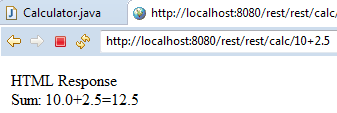# Java REST Web Service URI Parameters and RegexHere you can see how use custom URI parameters with and without a regular expression. With a calculator as example, that provides the four basic math operations as the web service methods, but using the desired math operator directly on the web service URI !

Let's use the Java REST Web Service with XML, JSON and HTML Response project as base, and do the calculator work this way:

<server URL><web service URI>/<number><math operator("+","-","*" or "/")><number>

Start keeping just the "SumHTML" method:

```@GET
@Path("/sum")
@Produces(MediaType.TEXT_HTML)
public String SumHTML(@QueryParam("x") int x,@QueryParam("y") int y) {
return "<html><body>HTML Response<br>Sum: " + x + "+" + y + "=" + (x+y) + "</body></html>";
}  ```

But changing to this:

```@GET
@Path("/{x}+{y}")
@Produces(MediaType.TEXT_HTML)
public String Sum(@PathParam("x") float x, @PathParam("y") float y) {
return "<html><body>HTML Response<br>Sum: " + x + "+" + y + "=" + (x+y) + "</body></html>";
}```

As we can see, the @Path annotation has the plus operator("+"), "x" and "y" variables, that will be used as the "Sum" function input float variables, which has the @PathParam annotation to get "x" and "y". That's it! now it's possible to access the method as below:Doing the same for the divide and multiply methods:

```@GET
@Path("/{x}*{y}")
@Produces(MediaType.TEXT_HTML)
public String Multiply(@PathParam("x") float x, @PathParam("y") float y) {
return "<html><body>HTML Response<br>Multiply: " + x + "*" + y + "=" + (x*y) + "</body></html>";
}

@GET
@Path("/{x}/{y}")
@Produces(MediaType.TEXT_HTML)
public String Divide(@PathParam("x") float x, @PathParam("y") float y) {
return "<html><body>HTML Response<br>Divide: " + x + "/" + y + "=" + (x/y) + "</body></html>";
}```

The URI parameters supports regular expressions too! and if the requested URI doesn't match the Regex pattern, a 404 error page will be shown by the server. So for the subtract method, "x" variable, let's allow just numbers with 2 digits, and 1 digit for the "y" variable:

```@GET
@Path("/{x: [0-9]{2}}-{y: [0-9]}")
@Produces(MediaType.TEXT_HTML)
public String Subtract(@PathParam("x") float x, @PathParam("y") float y) {
return "<html><body>HTML Response<br>Subtract: " + x + "-" + y + "=" + (x-y) + "</body></html>";
}```

That's all, the video below show us the result, first playing with the sum, multiply and divide methods, then playing with the subtract method and the Regex pattern: